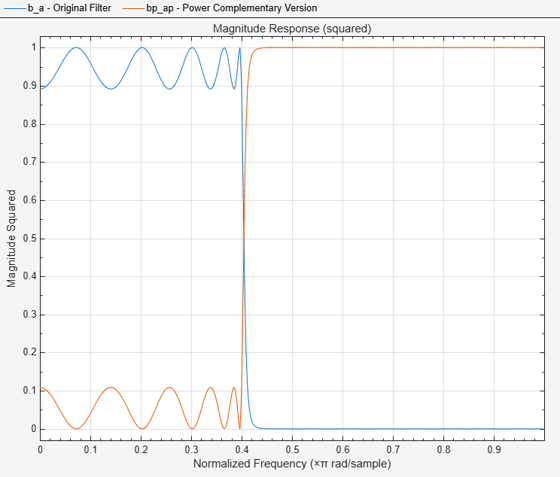# iirpowcomp

Power complementary IIR filter

## Syntax

``[bp,ap] = iirpowcomp(b,a)``
``[bp,ap] = iirpowcomp(b,a,c)``

## Description

example

````[bp,ap] = iirpowcomp(b,a)` returns the coefficients vectors `bp` and `ap`, of the power complementary IIR filter g(z) = bp(z) / ap(z), given the coefficients vectors `b` and `a` of the IIR filter h(z) = `b`(z)/ `a`(z) in . The vector `b` must be symmetric (Hermitian) or antisymmetric (antihermitian) and of the same length as the vector `a`. The two power complementary filters satisfy the relation|H(w)|2 + |G(w)|2 = 1.```
````[bp,ap] = iirpowcomp(b,a,c)` allow you to specify a complex scalar of unity magnitude, `c`, which forces `bp` to satisfy the generalized Hermitian property: `conj(bp(end:-1:1)) = c*bp````

## Examples

collapse all

Calaculate the coefficients of the power complementary IIR filter.

```[b,a]=cheby1(10,.5,.4); [bp,ap]=iirpowcomp(b,a);```

Compare the magnitude responses of the filters using FVTool.

```fvtool(b,a,bp,ap,'MagnitudeDisplay','Magnitude squared'); legend('Original Filter','Power Complementary Version');```## Input Arguments

collapse all

Numerator coefficients of the IIR filter, specified as a row vector. The vector b must be symmetric (Hermitian) or antisymmetric (antihermitian) and of the same length as the vector `a`.

Data Types: `single` | `double`
Complex Number Support: Yes

Denominator coefficients of the IIR filter, specified as a row vector.

Data Types: `single` | `double`
Complex Number Support: Yes

Complex scalar of unity magnitude, which forces the `bp` to satisfy generalized Hermitian property.

The generalized Hermitian property is given by:

`conj(bp(end:-1:1)) = c*bp`

When `c` is omitted, the function chooses `c` as follows:

• When `b` is real, the function chooses `c` as 1 or -1, whichever yields `bp` as real.

• When `b` is complex, `c` defaults to 1.

`ap` is always equal to `a`.

Data Types: `single` | `double`
Complex Number Support: Yes

## Output Arguments

collapse all

Numerator coefficients of the power complementary IIR filter, returned as a row vector.

Data Types: `single` | `double`

Denominator coefficients of the power complementary IIR filter, returned as a row vector.

Data Types: `single` | `double`
Complex Number Support: Yes

### Functions

Introduced in R2011a

## SupportGet trial now